Next: The Cailletaud single crystal Up: Materials Previous: Fiber reinforced materials.   Contents

### The Cailletaud single crystal model.

The single crystal model of Georges Cailletaud and co-workers  describes infinitesimal viscoplasticity in metallic components consisting of one single crystal. The orientations of the slip planes and slip directions in these planes is generally known and described by the normal vectors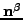and direction vectors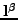, respectively, where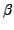denotes one of slip plane/slip direction combinations. The slip planes and slip directions are reformulated in the form of a slip orientation tensor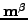satisfying: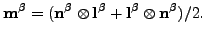(270)

The total strain is supposed to be the sum of the elastic strain and the plastic strain: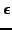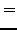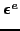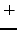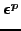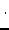(271)

In each slip plane an isotropic hardening variable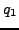and a kinematic hardening variable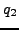are introduced representing the isotropic and kinematic change of the yield surface, respectively. The yield surface for orientationtakes the form: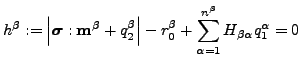(272)

where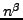is the number of slip orientations for the material at stake,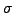is the stress tensor,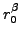is the size of the elastic range at zero yield andis a matrix of interaction coefficients. The constitutive equations for the hardening variables satisfy: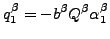(273)

and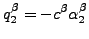(274)

where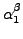and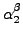are the hardening variables in strain space. The constitutive equation for the stress is Hooke's law: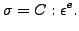(275)

The evolution equations for the plastic strain and the hardening variables in strain space are given by: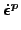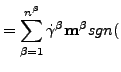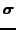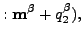(276)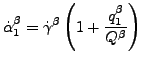(277)

and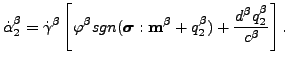(278)

The variable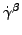is the consistency coefficient known from the Kuhn-Tucker conditions in optimization theory . It can be proven to satisfy: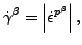(279)

where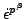is the flow rate along orientation. The plastic strain rate is linked to the flow rate along the different orientations by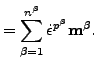(280)

The parameter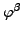in equation (278) is a function of the accumulated shear flow in absolute value through: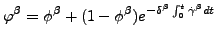(281)

Finally, in the Cailletaud model the creep rate is a power law function of the yield exceedance: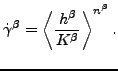(282)

The brackets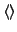reduce negative function values to zero while leaving positive values unchanged, i.e.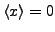if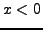and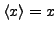if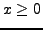.

In the present umat routine, the Cailletaud model is implemented for a Nickel base single crystal. It has two slip systems, a octaeder slip system with three slip directions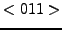in four slip planes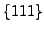, and a cubic slip system with two slip directionsin three slip planes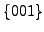. The constants for all octaeder slip orientations are assumed to be identical, the same applies for the cubic slip orientations. Furthermore, there are three elastic constants for this material. Consequently, for each temperature 21 constants need to be defined: the elastic constants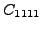,and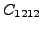, and a set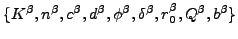per slip system. Apart from these constants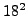interaction coefficients need to be defined. These are taken from the references  and assumed to be constant. Their values are included in the routine and cannot be influence by the user through the input deck.

The material definition consists of a *MATERIAL card defining the name of the material. This name HAS TO START WITH ''SINGLE_CRYSTAL'' but can be up to 80 characters long. Thus, the last 66 characters can be freely chosen by the user. Within the material definition a *USER MATERIAL card has to be used satisfying:

First line:

• *USER MATERIAL
• Enter the CONSTANTS parameter and its value, i.e. 21.

Following lines, in sets of 3:

First line of set:

•.
•.
•.
•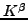(octaeder slip system).
•(octaeder slip system).
•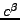(octaeder slip system).
•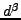(octaeder slip system).
•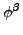(octaeder slip system).
Second line of set:
•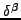(octaeder slip system).
•(octaeder slip system).
•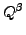(octaeder slip system).
•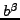(octaeder slip system).
•(cubic slip system).
•(cubic slip system).
•(cubic slip system).
•(cubic slip system).
Third line of set:
•(cubic slip system).
•(cubic slip system).
•(cubic slip system).
•(cubic slip system).
•(cubic slip system).
• Temperature.

Repeat this set if needed to define complete temperature dependence.

The crystal principal axes are assumed to coincide with the global coordinate system. If this is not the case, use an *ORIENTATION card to define a local system.

For this model, there are 60 internal state variables:

• the plastic strain tensor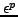(6)
• the isotropic hardening variables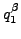(18)
• the kinematic hardening variables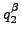(18)
• the accumulated absolute value of the slip rate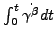(18)

These variables are accessible through the *EL PRINT (.dat file) and *EL FILE (.frd file) keywords in exactly this order (label SDV). The *DEPVAR card must be included in the material definition with a value of 60.

Example:

*MATERIAL,NAME=SINGLE_CRYSTAL
*USER MATERIAL,CONSTANTS=21
135468.,68655.,201207.,1550.,3.89,18.E4,1500.,1.5,
100.,80.,-80.,500.,980.,3.89,9.E4,1500.,
2.,100.,70.,-50.,400.
*DEPVAR
60


defines a single crystal with elastic constants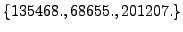, octaeder parameters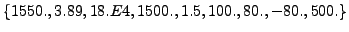and cubic parameters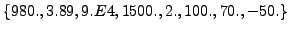for a temperature of 400.Next: The Cailletaud single crystal Up: Materials Previous: Fiber reinforced materials.   Contents
guido dhondt 2018-12-15